## a jeep start from the state of rest .if ots velocity became 60km/hr in 15 min. (i) what is the acceleration of the jeep?(ii) what is the

Question

a jeep start from the state of rest .if ots velocity became 60km/hr in 15 min. (i) what is the acceleration of the jeep?(ii) what is the distance coverd by jeep?​

in progress 0
2 months 2021-07-23T17:29:28+00:00 1 Answers 17 views 0

1. Explanation:

Here,

• Initial velocity (u) = 0 (as it starts from rest)
• Final velocity (v) = 60 km/h
• Time taken (t) = 15 min

Converting the quantities into its standard form :

→ v = 60 km/h

• 1 km/h = 5/18 m/s

→ v = ( 60 ×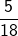) m/s

→ v = ( 10 ×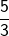) m/s

→ v =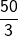m/s

v = 16.66 m/s

Also,

→ t = 15 minutes

→ t = (15 × 60) seconds

t = 900 seconds

### Calculatingacceleration:

v = u + at

• v is final velocity
• a is acceleration
• u is initial velocity
• t is time

→ 16.66 = 0 + 900a

→ 16.66 – 0 = 900a

→ 16.66 = 900a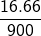= a

0.0185 m/s² = a

Acceleration is 0.0185 m/s².

## _________________________

### Calculatingdistancecovered:

v² u² = 2as

• v is final velocity
• a is acceleration
• u is initial velocity
• s is distance

→ (16.66)² – (0)² = 2 × 0.0185 × s

→ 277.5556 – 0 = 0.037s

→ 277.5556 = 0.037s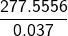= s

7501.50 m = s

Distance covered is 7501.50 m .